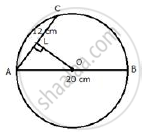Share

The Diameter and a Chord of a Circle Have a Common End-point. If the Length of the Diameter is 20 Cm and the Length of the Chord is 12 Cm, How Far is the Chord from the Centre of the Circle? - ICSE Class 10 - Mathematics

ConceptChord Properties - a Straight Line Drawn from the Center of a Circle to Bisect a Chord Which is Not a Diameter is at Right Angles to the Chord

Question

The diameter and a chord of a circle have a common end-point. If the length of the diameter is 20 cm and the length of the chord is 12 cm, how far is the chord from the centre of the circle?

SolutionAB is the diameter and AC is the chord.

Draw OL ⊥ AC
Since OL ⊥ AC and hence it bisects AC, O is the centre of the circle.
Therefore, OA = 10 cm and AL = 6 cm
Now, in Rt. ΔOLA,
AO^2 = AL^2  + OL^2
⇒ 10 ^2 = 6^2 + OL^2

⇒  OL^2  = 100  - 36 = 64
⇒ OL = 8 cm
Therefore, chord is at a distance of 8 cm from the centre of the circle.

Is there an error in this question or solution?

Video TutorialsVIEW ALL 

Solution The Diameter and a Chord of a Circle Have a Common End-point. If the Length of the Diameter is 20 Cm and the Length of the Chord is 12 Cm, How Far is the Chord from the Centre of the Circle? Concept: Chord Properties - a Straight Line Drawn from the Center of a Circle to Bisect a Chord Which is Not a Diameter is at Right Angles to the Chord.
S